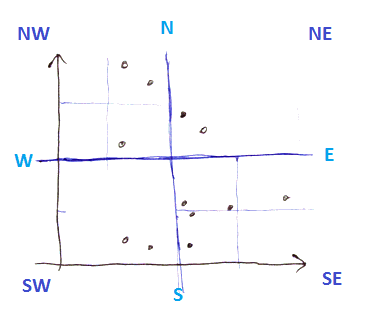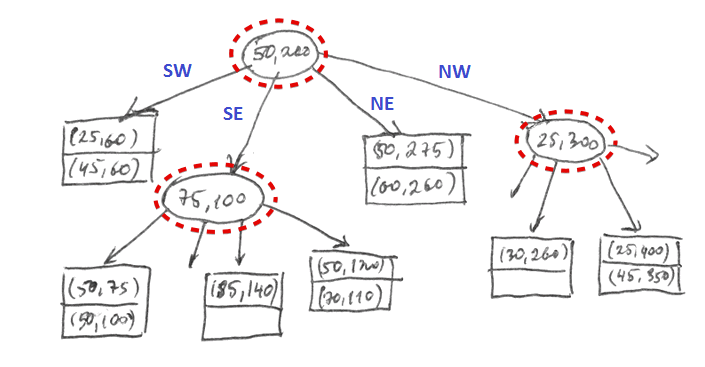# ML Wiki

## Contents

Idea:

• for $k$-dimensional space
• each node corresponds to a $k$-dimensional cube
• for 2D it's a square region

### BuildingAlgorithm

• if a number of points in a cube is larger that can fit in a block
• then we create an interior node
• and divide the cube recursively (i.e. we create 4 children for each quadrant)
• otherwise we create a leaf block## Operations

Lookups, Insertions and Deletions are very similar to kd-Trees